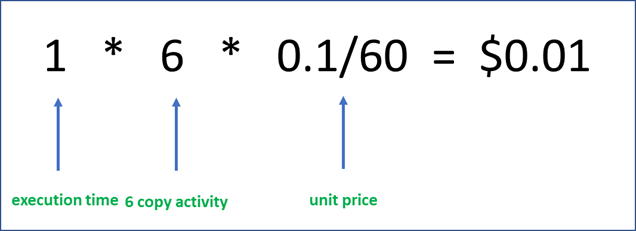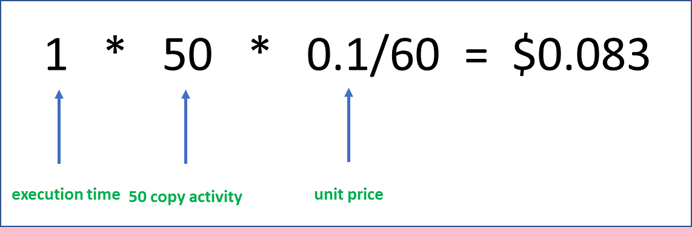# Examples for better understanding pricing model under different integration runtime types

APPLIES TO:Azure Data FactoryAzure Synapse Analytics

Tip

Try out Data Factory in Microsoft Fabric, an all-in-one analytics solution for enterprises. Microsoft Fabric covers everything from data movement to data science, real-time analytics, business intelligence, and reporting. Learn how to start a new trial for free!

In this article, we'll illustrate the pricing model using different integration runtime through some concrete examples. These examples only focus on copy activity, pipeline activity and external activity running on the integration runtime. It doesn't involve charges for Data Factory Pipeline Orchestration, Data Factory Operations and Data Factory Operations. For all pricing details, see Data Pipeline Pricing and FAQ.

The integration runtime, which is serverless in Azure and self-hosted in hybrid scenarios, provides the compute resources used to execute the activities in a pipeline. Integration runtime charges are prorated by the minute and rounded up.

Note

The prices used in this example below are hypothetical and are not intended to imply actual pricing.

## Azure integration runtime

Example 1: If there are 6 copy activities executed sequentially. 4 DIUs are used for the first 2 copy activities and the execution time of each is 40 seconds. The other 4 choose using 8 DIUs and the execution time of each is 1 minute and 20 seconds.

In this example, the execution time of the first 2 activities is both rounded up to 1 minute each. The other 4 are all rounded up to 2 minutes each.Example 2: If there are 50 copy activities triggered by Foreach. 4 DIUs are used for each copy activity, and each executes for 40 seconds. The parallel is configured as 50 in Foreach.

In this example, the execution time of each copy activity is rounded up to 1 minute. Although the 50 copy activities run in parallel, they use different computes. So they're charged independently.Example 3: If there are 6 HDInsight activities triggered by Foreach. The execution time of each is 9 minutes and 40 seconds. The parallel is configured as 50 in Foreach.

In this example, the execution time of each HDInsight activity is rounded up to 10 minutes. Although the 6 HDInsight activities run in parallel, they use different computes. So they're charged independently.## Azure integration runtime with managed virtual network enabled

Example 1: If there are 6 copy activities executed sequentially. 4 DIUs are used for each copy activity and the execution time of each is 40 seconds. Assume the queue time is 1 minute and no TTL enabled.

In this example, the execution time and queue time totally are rounded up to 2 minutes. As no TTL is enabled, every copy activity has a queue time. The price for copy activity using Azure integration runtime with managed virtual network enabled is \$0.25 /DIU-hour.Example 2: If there are 6 copy activities executed sequentially. 4 DIUs are reserved for copy activity and the execution time of each is 40 seconds. Assume the queue time is 1 minute and TTL is 5 minutes.

As the compute is reserved, the 6 copy activities aren't rounded up independently and are charged together. And TTL is enabled, so only the first copy activity has queue time.Example 3: If there are 6 HDInsight activities triggered by Foreach. The execution time of each is 9 minutes and 40 seconds. The parallel is configured as 50 in Foreach. Compute size is 1. TTL is 30 minutes.

In this example, the execution time of each HDInsight activity is rounded up to 10 minutes. As the 6 HDInsight activities run in parallel and within the concurrency limitation (800), they're only charged once.## Self-hosted integration runtime

Example 1: If there are 6 copy activities executed sequentially. The execution time of each is 40 seconds.

In this example, the execution time of each copy activity is rounded up to 1 minute each.Example 2: If there are 50 copy activities triggered by Foreach. The execution time of each is 40 seconds. The parallel is configured as 50 in Foreach.

In this example, the execution time of each copy activity is rounded up to 1 minute. Although the 50 copy activities run in parallel, they're charged independently.Example 3: If there are 6 HDInsight activities triggered by Foreach. The execution time of each is 9 minutes and 40 seconds. The parallel is configured as 50 in Foreach.

In this example, the execution time of each HDInsight activity is rounded up to 10 minutes. Although the 6 HDInsight activities run in parallel, they're charged independently.Now that you understand the pricing for Azure Data Factory, you can get started!